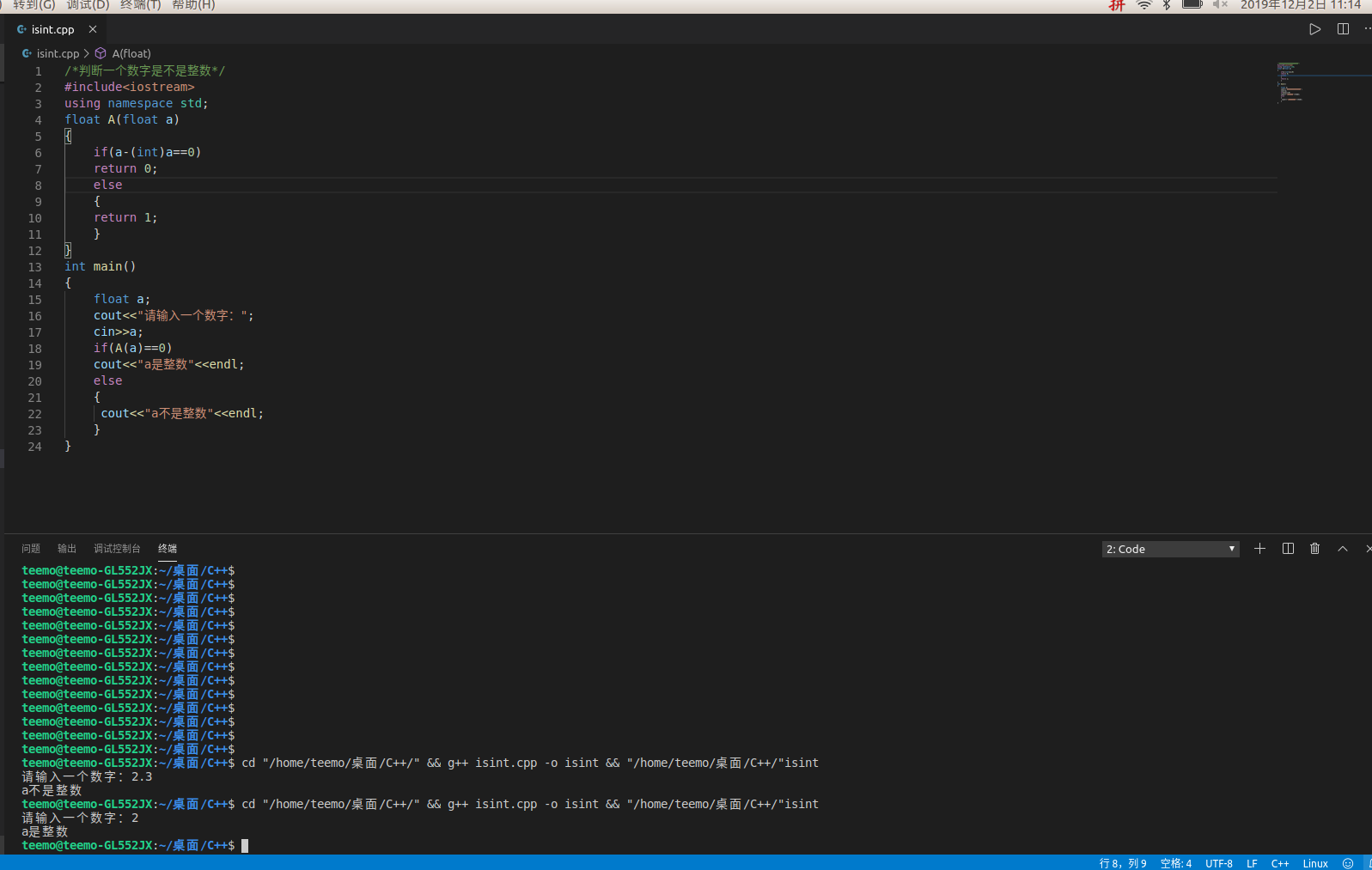• c++判断是不是整数
万次阅读
2016-02-04 18:20:06

运行下面的代码

#include <iostream>
#include <cmath>
using namespace std;

int main()
{
int n;
while(cin>>n) {
double result = log(n)/log(3);
cout<<result<<endl;
cout<<(int)result<<endl;
}
return 0;
}


输入253，结果输出

5
4


怀疑由于计算精度丢失所以result并不是5而是小于且很接近5的一个数，但输入的时候显示5，因此取整数部分为4。

下面利用代码验证一下

#include <iostream>
#include <cmath>
using namespace std;

int main()
{
double n;
while(cin>>n) {
cout<<n<<endl;
}
return 0;
}


输入4.99999999999999
结果输出5。说明那个result的确是一个小于5但很接近5的数。

将代码改一下，就能判断一个double类型的数是否整数
#include <iostream>
#include <cmath>
using namespace std;

int main()
{
double n;
while(cin>>n) {
if(abs(round(n) - n) < 0.000000000000001) {
cout<<"is an integer"<<endl;
} else {
cout<<"not is an integer"<<endl;
}
}
return 0;
}

更多相关内容
• C++编程第一步：如何判断一个数字是不是整数? 代码如下： #include<iostream> using namespace std; float A(float a) { if(a-(int)a==0) return 0; else { return 1; } } int main() { float a; c.....

C++编程第一步：如何判断一个数字是不是整数?
代码如下：

#include<iostream>
using namespace std;
float A(float a)
{
if(a-(int)a==0)
return 0;
else
{
return 1;
}
}
int main()
{
float a;
cout<<"请输入一个数字：";
cin>>a;
if(A(a)==0)
cout<<"a是整数"<<endl;
else
{
cout<<"a不是整数"<<endl;
}
}over~

展开全文• 2、判断整数还是小数：定义接收内容的字符串bai string s，调用C++的getline方法，格式如下：getline(cin，s)，getline可以接收空格，遇到换行zhi输入结束。然后依次判断字符串中的字符，是否为数字，如果遇到一个非...

1、判断是整数还是字符：通过int n = scanf("%d",&a); 判断n的值，然后，如果要继续输入，用flush()函数，清晰缓冲区，再次输入。
1.1、对于C++来说，使用cin输入，如果cin输入出错，会返回false, 然后，使用cin.clear()函数清空缓存区，继续输入。clear() 类似C中的flush。
2、判断整数还是小数：定义接收内容的字符串string s，调用C++的getline方法，格式如下：getline(cin，s)，getline可以接收空格，遇到换行输入结束。然后依次判断字符串中的字符，是否为数字，如果遇到一个非数字的字符，则不是整数。

void test()
{
string str1;
cin >> str1;
for (size_t i = 0; i < str1.size(); i++)
{
switch (str1.at(i))
{
case '0':
case '1':
case '2':
case '3':
case '4':
case '5':
case '6':
case '7':
case '8':
case '9': break;
default: cout << "有小数" << endl;
break;
}
}
}


最后，一般判断之后需要转换为原来的样子：使用自带的字符数组转换方式：

// array to integer
to_price=atoi(price.c_str());
// 类似还有atof atol atoll
// 反转 to_string()


整数变成字符串直接使用to_string(int val);
但是，float a=25.3, 使用to_string(a), 之后输出的字符串a为25.299999；
float a=25.4, 之后输出的字符串a为25.400000；
so:
(1) 注意小数转换为字符时，加上的0000；
(2) 注意小说后为奇数的数据丢失情况。

// 控制字符串显示的长度
cout.write(str.c_str(), 5);


// 或者使用double modf(integer , fptr)函数
<math.h>
double modf(double x,double * ip)
把x分成整数和小数两部分，两部分正负与x相同，函数返回小数部分，整数部分保存在
ip中
函数名: modf
功 能: 把数分为整数和小数 （The modf function breaks down the floating-point value x into fractional and integer parts, each of which has the same sign as x. The signed fractional portion of x is returned. The integer portion is stored as a floating-point value at intptr.

用 法: double modf(double x, double *intptr);
程序例:

#include <math.h>
#include <stdio.h>
int main(void)
{
double fraction, integer;
double number = 100000.567;
fraction = modf(number, &integer);
printf("The whole and fractional parts of %lf are %lf and %lf\n",
number, integer, fraction);
return 0;
}


展开全文• #include<stdio.h> #include<math.h> bool compare(float f) { int x = f; float temp = f - x; /*if (temp > -(1e-6) && temp < 1e-6) return true;... bool t=compa
#include<stdio.h>
#include<math.h>
bool  compare(float f)
{
int x = f;
float temp = f - x;
/*if (temp > -(1e-6) && temp < 1e-6)
return true;*/
if(fabs(temp)<1e-6)
return true;
return false;
}
int main()
{
bool t=compare(-3.00001);
t = compare(3.1);
t = compare(3.0000000001);
return 0;
}

展开全文• bool isDigit(char a) { if (a - '0' == 0 || a - '0' == 1 || a - '0' == 2 || a - '0' == 3 || a - '0' == 4 || a...i++)//字符串内容是否为整数 if (!isDigit(y[i])) { cout输入内容不是整数......"; return; }
• 判断整数是否既是5又是7的整数倍 题目描述 输入一个正整数判断该数是否既是5又是7的整数倍。若是，则输出Yes，否则输出No. 输入 输入一个正整数 输出 若该数是5和7的倍数，则输出Yes，否则输出No. 样例输入 Copy...
• C++判断用户输入是否为正整数整数 判断用户输入是否为正整数 不能输入小数 不能输入字母、单词 不能输入文字 不能输入负数 代码： 在这里插入代码片字符串
• 定义一个函数f来判断整数的位数，用循环来实现。对一个大于0的int整数除10，每次做除法，计数器就+1，直至整数位为0。计数器t所得到的便是这个整数的位数。 #include<iostream> using namespace std; int f...
• 最近遇到一个小问题，判断输入的内容是否为整数。具体方法如下：首先定义接收内容的字符串 string s;调用C++的 getline方法，格式如下：getline(cin，s)，getline可以接收空格，遇到换行输入结束。然后依次判断字符...
• #include <iostream> using namespace std; int main() { long long int a,b=0;//这里的定义看情况而定. cout<<"请输入一个数："; cin>>a; while(a!=0) { b++; a=a/10;...e...
• ## C++如何检查浮点数是否为整数

千次阅读 多人点赞 2020-04-16 19:38:56
如何检查浮点数是否为整数 今天在写程序的时候，突然遇到这个问题——如何判断一个浮点数小数点后是否有小数值？ 刚开始想了一个比较麻烦的方法，感觉不妥，太浪费时间了，然后就上网看了一些其他人的思路，借此...
• 主要为大家详细介绍了C++实现正整数的四则运算表达式，文中示例代码介绍的非常详细，具有一定的参考价值，感兴趣的小伙伴们可以参考一下
• C++ 判断字符串是否是整数或浮点数 在实际写代码过程中，我们经常需要知道一串字符串是否为整数或是浮点数，当然，若是用C++自带的stream等，当然可以非常方便的判断，但效率并不高。因此，我们需要编写出对应的函数...字符串
• 输入一个正整数判断是不是素数（C++） 设和和我一样的初学者看。
• 直接上码 #include <stdio.h>...// 判断一个字符串是为非负整数 正确返回数字长度, 错误返回0 int is_nonnegative_int(char* str); int main() { int ret = 0; if(ret = is_nonnegative_i
• 没啥好描述的，就是一个简单的c++小代码而已，需要的可以参考下
• 思路：判断一个整数m是否是素数，只需把 m 被 2 ~ m-1 之间的每一个整数去除，如果都不能被整除，那么 m 就是一个素数。 #include using namespace std; int main() { int p; cin >> p; bool f = 0; if (p == 1) { f...
• 判断整数是正整数、负整数还是零 题目描述 输入一个整数判断该数是正整数、负整数还是零。 输入 输入一个整数。 输出 如果是正整数，输出’+’；如果是负整数，输出’-’；如果是零，输出’0’。 样例输入 -360 ...
• //输出0，是不整数 float a = 3; int b = 3; printf("%d\n", a==b); //输出1 printf("%d\n", 3.0==3); //输出1 printf("%d\n", 3.000000001==3);//输出0 printf("%d\n", 3.0000000000000000000000001==3);//...
• 详见： ...tags=&title=&difficulty=0&judgeStatus=0&rp=0 质数是只能被1及其本身整除的正整数。例如2是质数：2÷1=2，2÷2=1，即2被...判断一个数n是不是质数， 1.首先，任何正整数都能被1及其本身n整除算法 动态规划
• ## python判断整数

千次阅读 2020-11-20 20:03:47
python输出整数的方法：先使用str()函数将数字转换成字符串赋值给变量i，再用“if i.count(‘.’) == 0”语句判断字符串中是否没有小数点，如果是则输出这个字符串，这样输出的数字就都是整数了示例代码如下：x = ...
• #include <iostream> #include <cstring> #include <iomanip> using namespace std; int main(){ char ch; cin>>ch; int i,j,y=0; for(i=0;i<strlen(ch)-1;...j&...
• bool is_prime(int n){ for(int i=2;i*i<=n;i++){ if(n%i==0) return false; } return n!=1; }
• [TIPS] 判断一个数为整数还是小数 | C++ 判断一个数是整数还是小数，代码如下： bool A(float a) { if(a-(int)a==0) //核心在此a-(int)a return 0; else return 1; }
• C/C++ 判断两个整数相乘是否溢出 判断两int相乘是否溢出，目前找到的最正确方式： int is_mul_overflow(int a, int b) { if( a >= 0 && b >=0 ) { return INT_MAX / a < b; } else if( a...
• 正在学习编程中，有错误请指出 ...//判断输入整数是否为回文数 int main() { int X; cout << "请输入一个整数:"; cin >> X; int temp = 0; int Y = 0; int Z = X; while (X > 0) {
• 判断一个整数是否为2的幂次方法有以下几种： 1.循环除2 这是最简单最好理解的方式。对于一个数如果是2的幂次，则其肯定可以被2一直整除直到其值为1。 所以可以通过一个while循环判断： void judge(int b) { while ...c语言
• 输入有两行：第一行包含一个正整数k，第二行包含k个正整数，每两个正整数用一个空格分开。 输出 输出有三行，第一行为1出现的次数，，第二行为5出现的次数，第三行为10出现的次数。 样例输入Copy 5 1 5 8 10 5 ......

# c++判断是不是整数c++ 订阅